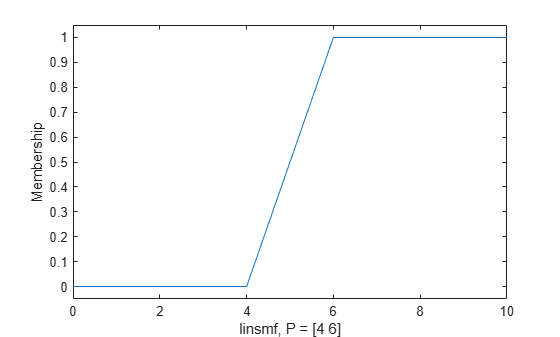# linsmf

Linear s-shaped saturation membership function

Since R2022a

## Syntax

``y = linsmf(x,params)``

## Description

This function computes fuzzy membership values using a linear s-shaped membership function. You can also compute this membership function using a `fismf` object. For more information, see fismf Object.

This membership function is related to the `trimf`, `trapmf`, and `linzmf` membership functions.

example

````y = linsmf(x,params)` returns fuzzy membership values computed using a linear s-shaped saturation membership function.When a < b:$f\left(x;a,b\right)=\left\{\begin{array}{ll}0,\hfill & xb\hfill \end{array}\right\}$When a = b:$f\left(x;a,b\right)=\left\{\begin{array}{cc}0,& xTo define the membership function parameters, specify `params` as the vector [a b].Membership values are computed for each input value in `x`.```

## Examples

collapse all

Specify input values across the universe of discourse.

`x = 0:0.1:10;`

Evaluate a linear s-shaped membership function for the input values.

`y = linsmf(x,[4 6]);`

Plot the membership function.

```plot(x,y) xlabel('linsmf, P = [4 6]') ylabel('Membership') ylim([-0.05 1.05])```## Input Arguments

collapse all

Input values for which to compute membership values, specified as a scalar or vector.

Membership function parameters, specified as the vector [a b]. Parameter a defines the foot of the membership function, and b defines its shoulder. Setting a equal to b produces a crisp membership function.

## Output Arguments

collapse all

Membership value, returned as a scalar or a vector. The dimensions of `y` match the dimensions of `x`. Each element of `y` is the membership value computed for the corresponding element of `x`.

## Alternative Functionality

### `fismf` Object

You can create and evaluate a `fismf` object that implements the `linsmf` membership function.

```mf = fismf("linsmf",P); Y = evalmf(mf,X);```

Here, `X`, `P`, and `Y` correspond to the `x`, `params`, and `y` arguments of `linsmf`, respectively.

## Version History

Introduced in R2022a